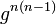# N-abelian implies n(n-1)-central

## Statement

Suppose$G$ is a group and$n$ is an integer other than 0 or 1. Suppose that$G$ is a n-abelian group, i.e., the map$x \mapsto x^n$ is an endomorphism of$G$ (hence, it is a universal power endomorphism). Then,$G$ is n(n-1)-central: the inner automorphism group of$G$ has exponent dividing$n(n-1)$.

## Related facts

### Converse

We say that a group is a n-abelian group if the$n^{th}$ power map is an endomorphism. Here are some related facts about$n$-abelian groups.

Value of$n$ (note that the condition for$n$ is the same as the condition for$1-n$) Characterization of$n$-abelian groups Proof Other related facts
0 all groups obvious
1 all groups obvious
2 abelian groups only 2-abelian iff abelian endomorphism sends more than three-fourths of elements to squares implies abelian
-1 abelian groups only -1-abelian iff abelian
3 3-abelian group means: 2-Engel group and derived subgroup has exponent dividing three Levi's characterization of 3-abelian groups cube map is surjective endomorphism implies abelian, cube map is endomorphism iff abelian (if order is not a multiple of 3), cube map is endomorphism implies class three
-2 same as for 3-abelian (based on n-abelian iff (1-n)-abelian)

## Facts used

1. n-abelian implies every nth power and (n-1)th power commute

## Proof

Given: A group$G$ such that the map$x \mapsto x^n$ is an endomorphism of$G$. Elements$g,h \in G$ (possibly equal).

To prove:$g^{n(n-1)}$ commutes with$h$. This is sufficient because$h$ being arbitrary, it shows that$g^{n(n-1)}$ is central, and$g$ being arbitrary, it shows that$G/Z(G)$ has exponent dividing$n(n-1)$.

Proof:

Step no. Assertion/construction Facts used Given data used Previous steps used Explanation
1$g^{n(n-1)}$ commutes with$h^n$. Fact (1) The map$x \mapsto x^n$ is an endomorphism of$G$. By the given data and Fact (1), every$n^{th}$ power commutes with every$(n-1)^{th}$ power. Treating$g^{n(n-1)} = (g^n)^{n-1}$ as a$(n-1)^{th}$ power and$h^n$ as a$n^{th}$ power, we obtain that$g^{n(n-1)}$ commutes with$h^n$.
2$g^{n(n-1)}$ commutes with$h^{n-1}$. Fact (1) The map$x \mapsto x^n$ is an endomorphism of$G$. By the given data and Fact (1), every$n^{th}$ power commutes with every$(n-1)^{th}$ power. Treating$g^{n(n-1)} = (g^{n-1})^n$ as a$n^{th}$ power and$h^{n-1}$ as a$(n-1)^{th}$ power, we obtain that$g^{n(n-1)}$ commutes with$h^{n-1}$.
3 The centralizer in$G$ of$g^{n(n-1)}$ contains the elements$h^n$ and$h^{n-1}$. Since the centralizer of any element is a subgroup, it also contains the quotient$h^n(h^{n-1})^{-1} = h$, and hence$g^{n(n-1)}$ commutes with$h$. Steps (1), (2) The first part follows from Steps (1) and (2). The rest is self-explanatory.